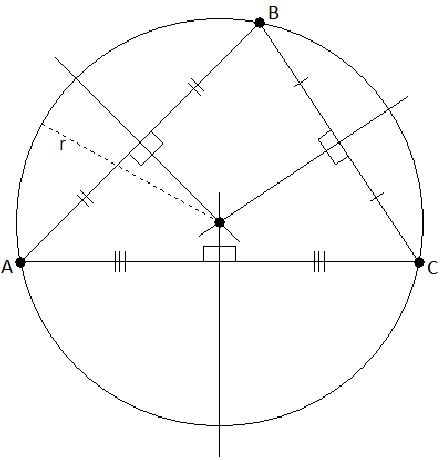# Programming contests

## DEIK Regionális Programozó Csapatverseny, egyetemi/főiskolai kategória, 2015. november 29.

November 29, 2015 10:40 AM – November 29, 2015 3:40 PM

# Circle Through Three Points

Your are to write a program that, given the Cartesian coordinates of three points on a plane, will find the equation of the circle through them all. The three points will not be on a straight line.

The solution is to be printed as two equations. The form of the first equation is the following:

(x – h)2 + (y – k)2 = r2

The second equation should look like this:

x2 + y2 + cx + dy + e = 0## Input Specification

Each line of input to your program will contain the x and y coordinates of three points, in the order Ax, Ay, Bx, By, Cx, Cy. These coordinates will be real numbers separated from each other by one or more spaces.

## Output Specification

Your program must print the required equations on two lines using the format given in the sample below. Your computed values for h, k, r, c, d, and e in the equations above are to be printed with three digits after the decimal point. Plus and minus signs in the equations should be changed as needed to avoid multiple signs before a number. Plus, minus, and equal signs must be separated from the adjacent characters by a single space on each side. No other spaces are to appear in the equations. If the value of h or k is 0 in the first equation, then this value, the preceding operator, and the associated parentheses should be omitted. Similarly, if the value of c, d, or e is 0 in the second equation, then the respective term and the preceding operator should be omitted. Print a single blank line after each equation pair.

## Sample Input

1. `7.0 -5.0 -1.0 1.0 0.0 -6.0`
2. `1.0 7.0 8.0 6.0 7.0 -2.0`
download as text file

## Output for Sample Input

1. `(x - 3.000)^2 + (y + 2.000)^2 = 5.000^2`
2. `x^2 + y^2 - 6.000x + 4.000y - 12.000 = 0`
3. `(x - 3.921)^2 + (y - 2.447)^2 = 5.409^2`
4. `x^2 + y^2 - 7.842x - 4.895y - 7.895 = 0`
download as text file
University of Debrecen; Faculty of Informatics; v. 03/01/2019